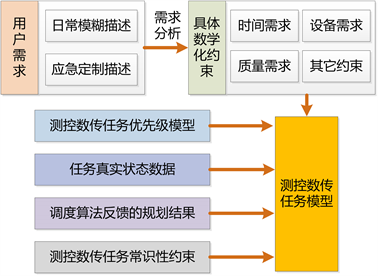#### 期刊菜单

A CSOP-Based Static Scheduling of Low-Orbit Satellite Measurement and Control Data Transmission Resources Model
DOI: 10.12677/JAST.2021.93011, PDF, HTML, XML, 下载: 178  浏览: 340

Abstract: Aiming at the problem of low-orbit satellite measurement and control data transmission resource scheduling, a static scheduling model based on the constraint satisfaction optimization problem (CSOP) is proposed. By analyzing the principle and characteristics of multi-satellite measurement and control data transmission resource scheduling, the related concepts are defined and standardized, and the modeling of the object and the scheduling process is realized. In this paper, the process of assigning measurement and control data transmission resources to spacecraft measurement and control data transmission tasks and selecting a suitable time window for the spacecraft are regarded as equivalent processes. The number of measurement and control data transmissions and the measurement and control data transmission duration in the visible circle of measurement and control data transmission resources are used by the spacecraft. Time describes its task requirements. Based on the standardized description of measurement and control requirements, data transmission requirements and constraints, a CSOP-based six-tuple static scheduling model is constructed.

1. 引言

2. CSP和CSOP概念

2.1. 约束满足问题CSP概念

2.2. 约束满足优化问题CSOP概念

3. 基于CSOP的低轨卫星测控数传资源静态调度模型Figure 1. Model composition of telemetry control and data transmission mission

3.1. 任务集合

$A=\left\{{w}_{1,1}^{p,q},\cdots ,{w}_{1,{N}_{1}}^{p,q};\cdots ;{w}_{i,1}^{p,q},\cdots ,{w}_{i,{N}_{i}}^{p,q};\cdots ;{w}_{n,1}^{p,q},\cdots ,{w}_{n,{N}_{n}}^{p,q}\right\}$

${N}_{0}=0$${K}_{0}=0$${K}_{i}=\left\{k|\underset{j=0}{\overset{j=i-1}{\sum }}{N}_{j}$i\in \left\{1,\cdots ,n\right\}$

3.2. 资源集合

$S=\left\{{s}_{1,1},\cdots ,{s}_{1,{L}_{1}};\cdots ;{s}_{f,1},\cdots ,{s}_{f,{L}_{f}};\cdots ;{s}_{l,1},\cdots ,{s}_{l,{L}_{l}}\right\}$

3.3. 可见弧段集合

3.4. 决策变量集合

1) 任务需求规划变量

2) 地基资源选择决策变量

3.5. 约束集合

3.5.1. 任务需求约束

1) 指定圈次测控任务需求

${t}_{k}^{p,q}=1$

2) 低轨卫星长期管理任务基本需求

$\underset{k\in {\Omega }_{0}}{\sum }{t}_{k}^{p,q}={P}_{0}$

$\underset{k\in {\Omega }_{1}}{\sum }{t}_{k}^{p,q}={P}_{1}$

3) 数传任务需求

$\underset{k\in {\Omega }_{3}}{\sum }{t}_{k}^{p,q}=Q$

3.5.2. 决策变量约束

$\underset{h}{\sum }\text{\hspace{0.17em}}{x}_{k,h}\le \underset{q\in \left\{0,1\right\}}{\sum }{t}_{k}^{p,q}$

3.5.3. 时间窗口约束

$st{w}_{k,h}\ge st{w}_{{k}^{\prime },h}$ 时，

${x}_{k,h}\cdot st{w}_{k,h}-{x}_{{k}^{\prime },h}\left(et{w}_{{k}^{\prime },h}+{r}_{h}\right)+\left(1-{x}_{k,h}\right)\cdot M\ge 0$

$st{w}_{k,h}\le st{w}_{{k}^{\prime },h}$ 时，

${x}_{{k}^{\prime },h}\cdot st{w}_{{k}^{\prime },h}-{x}_{k,h}\left(et{w}_{k,h}+{r}_{h}\right)+\left(1-{x}_{{k}^{\prime },h}\right)\cdot M\ge 0$

1) 任务需求约束： ${C}_{1}\vee \left({C}_{2}\wedge {C}_{3}\right)\vee {C}_{4}$

2) 决策变量约束： ${C}_{5}\vee {C}_{6}$

3) 时间窗口约束： ${C}_{7}$

3.6. 优化目标

$J=\mathrm{max}\underset{k}{\sum }\left(\underset{h}{\sum }\text{\hspace{0.17em}}{x}_{k,h}\cdot {P}_{k}\right)$

3.7. 小结

4. 结论

  Arbabi, M. (1984) Range Scheduling Automation. IBM Technical Directions, 10, 57-62.  Gooley, T.D. (1993) Automating the Satellite Range Scheduling Process. Air Force Institute of Technology, Ohio.  Parish, S.A. (1994) A Genetic Algorithm Approach to Automating Satellite Range Scheduling. Air Force Institute of Technology, Ohio.  Schalck (1993) Automating Satellite Range Scheduling. Air Force Institute of Technology, Ohio.  Jang, K. (1996) The Capacity of the Air Force Satellite Control Network. Air Force Institute of Technology, Ohio.  Burrowbridge, S. (1999) Optimal Allocation of Satellite Network Resources. Virginia Polytechnic Institute and State University, Virginia.  王远振, 高卫斌, 聂成. 多星地面站系统资源配置优化研究综述[J]. 系统工程与电子技术, 2004, 26(4): 437-439.  冯宏胜. 卫星地面站测控资源配置建模与优化方法[D]: [硕士学位论文]. 长沙: 国防科技大学, 2010.  常飞. 卫星地面站数传资源配置优化模型与算法研究[D]: [博士学位论文]. 长沙: 国防科技大学, 2010.